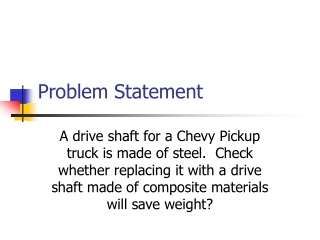DownloadDownload PresentationProblem Statement

# Problem Statement

Télécharger la présentation## Problem Statement

- - - - - - - - - - - - - - - - - - - - - - - - - - - E N D - - - - - - - - - - - - - - - - - - - - - - - - - - -
##### Presentation Transcript

1. Problem Statement A drive shaft for a Chevy Pickup truck is made of steel. Check whether replacing it with a drive shaft made of composite materials will save weight?

2. Design of a Composite Drive Shaft

3. Why composite materials? • Light weight___ reduces energy consumption. • Fatigue resistant___ durable life. • Non-corrosive___ reduced maintenance cost and increased life. • Single piece___ reduces manufacturing cost.

4. Problem Description Design Constraints 1. Maximum horsepower= 175 HP @4200rpm 2. Maximum torque = 265 lb-ft @2800rpm 3. Factor of safety = 3 3. Outside radius = 1.75 in 4. Length = 43.5 in

5. Torque in Drive Shaft In first gear - the speed is 2800 rpm (46.67 rev/s) - ground speed of 23 mph (405 in/sec) Diameter of tire = 27 in Revolutions of tire = = 4.78 rev/s Differential ratio = 3.42 Drive shaft speed = 4.78 x 3.42 = 16.35 rev/s Torque in drive shaft = = 755 lb-ft

6. Maximum Frequency of Shaft Maximum Speed = 100 mph Drive Shaft Revs = 16.35 rev/s at 23 mph Maximum Frequency= = 71.1 Hz

7. Design Parameters • Torque Resistance. • Should carry load without failure • Not rotate close to natural frequency. • Need high natural frequency otherwise whirling may take place • Buckling Resistance. • May buckle before failing

8. Steel Shaft – Torque Resistance Shear Strength, max = 25 Ksi Torque, T = 755 lb-ft Factor of Safety, FS = 3 Outer Radius, c = 1.75 in Polar moment of area, J = cin =1.69 in t = 1.75-1.69 = 0.06 in = in

9. Steel Shaft - Natural Frequency fn = Acceleration due to gravity, g=32.2 ft/s2 Young’s modulus, E = 30 Msi Mass per unit length, W = 0.19011 lb/in Length, L = 43.5 in Second Moment of Area, I = fn = 204 Hz (meets minimum of 71.1Hz)

10. Steel Shaft - Torsional Buckling T= Mean raduis, r = 1.6875 in Thickness, t = 1/16 in Young’s modulus, E = 30 Msi Critical Buckling Load, T = 5519 lb-ft (Meets minimum of 755 lb-ft)

11. Designing with a composite

12. Load calculations for PROMAL Nxy = T = 755 lb-ft r = 1.75 in Nxy = 470.8 lb / in

13. Composite Shaft-Torque Resistance Inputs to PROMAL: Glass/Epoxy from Table 2.1 Lamina Thickness = 0.0049213 in Stacking Sequence: (45/-45/45/-45/45)s Load Nxy = 470.8 lb / in Outputs of PROMAL: Smallest Strength Ratio = 4.1 Thickness of Laminate: h = 0.0049213*10 = 0.04921 in

14. Composite Shaft - Natural Frequency fn = g = 32.2 ft/s2 Ex = 1.821 Msi I = 0.7942 in4 W = 0.03999 L = 43.5 in Hence fn = 98.1 Hz (meets minimum 71.1 Hz)

15. Composite Shaft - Torsional Buckling T = rm = 1.75 - = 1.72539 in t = 0.04921 in Ex = 1.821 Msi Ey = 1.821 Msi T = 183 lb-ft (does not meet 755 lb-ft torque)

16. Comparison of Mass